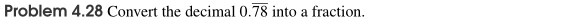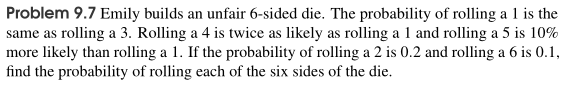# Math Challenge I-C Textbooks

Please see the sections below for answer keys, topics covered, and sample problems for each of the MC I-C textbooks.

### Algebra

The answer key for MC I-C Algebra is available by clicking here.

#### Chapter Topics

1. Introduction to Algebra and Solving Equations
2. Solving Inequalities
3. Absolute Values
4. Intro to Functions and Linear Functions
5. Problem Solving with Linear Functions
6. Systems of Linear Equations
7. Problem Solving with Systems of Equations
9. Problem Solving with Exponents and Radicals
10. Review with Math Contest Problems

#### Sample Questions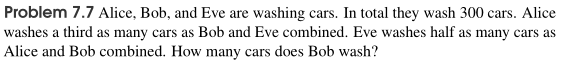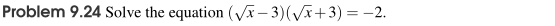### Topics in Algebra

The answer key for MC I-C Topics in Algebra is available by clicking here.

#### Chapter Topics

1. Polynomials and Factoring
2. Factoring Formulas and Patterns
6. Modeling and Problem Solving with Functions
7. Solving Equations with Square Roots
8. Rational Expressions and Polynomial Long Division
9. Solving Rational Equations
10. Math Contest Review

#### Sample Questions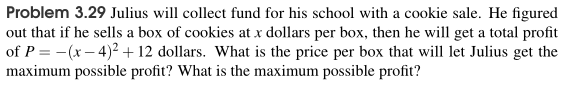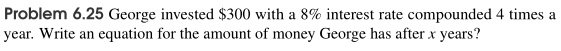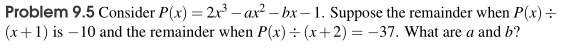### Geometry

The answer key for MC I-C Geometry is available by clicking here.

#### Chapter Topics

1. Parallel Lines and Into to Coordinates
2. Measurements: Areas and Lengths
3. Congruent and Similar Triangles
4. Right Triangles and Basic Trigonometry
5. Angles and Areas with Polygons
6. Circles
7. Intro to 3-D Geometry
8. Solids in 3-D Geometry
9. Conic Sections
10. Math Contest Review

#### Sample Questions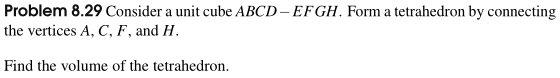### Finite Math

The answer key for MC I-C Finite Math is available by clicking here.

#### Chapter Topics

1. Numbers, Place Values, and Divisibility
2. Primes, Factors, and Multiples
3. Remainders and Modular Arithmetic
4. Sequences And Series
5. Recursive Sequences
6. Factorials, Permutations, and Combinations
7. Sets and Functions
8. Bijections and Stars and Bars
9. Probability
10. Math Contest Review

#### Sample Questions